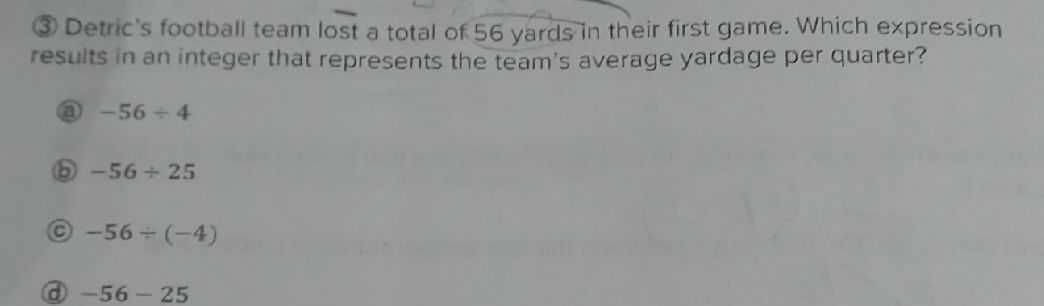### Still have math questions?

Arithmetic
Question(3) Detric's football team lost a total of $$56$$ yards in their first game. Which expression results in an integer that represents the team's average yardage per quarter?

(a) $$- 56 \div 4$$

(b) $$- 56 \div 25$$

(c) $$- 56 \div ( - 4 )$$

(d) $$- 56 - 25$$